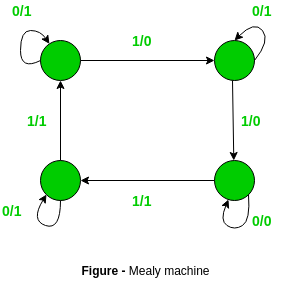# Difference between Mealy machine and Moore machine

• Difficulty Level : Easy
• Last Updated : 02 Nov, 2020

Prerequisite – Mealy and Moore Machines
Mealy Machine – A mealy machine is defined as a machine in theory of computation whose output values are determined by both its current state and current inputs. In this machine atmost one transition is possible.
It has 6 tuples: (Q, q0, ∑, O, δ, λ’)
Q is finite set of states
q0 is the initial state
∑ is the input alphabet
O is the output alphabet
δ is transition function which maps Q×∑ → Q
‘λ’ is the output function which maps Q×∑→ O

Diagram –Moore Machine – A moore machine is defined as a machine in theory of computation whose output values are determined only by its current state.
It has also 6 tuples: (Q, q0, ∑, O, δ, λ)
Q is finite set of states
q0 is the initial state
∑ is the input alphabet
O is the output alphabet
δ is transition function which maps Q×∑ → Q
λ is the output function which maps Q → O

Diagram –Moore Machine –

1. Output depends only upon present state.

2. If input changes, output does change.

3. More number of states are required.

4. There is less hardware requirement for circuit implementation.

5. They react slower to inputs(One clock cycle later).

6. Synchronous output and state generation.

7. Output is placed on states.

8. Easy to design.

Mealy Machine –

1. Output depends on present state as well as present input.

2. If input changes, output also changes.

3. Less number of states are required.

4. There is more hardware requirement for circuit implementation.

5. They react faster to inputs.

6. Asynchronous output generation.

7. Output is placed on transitions.

8. It is difficult to design.

My Personal Notes arrow_drop_up